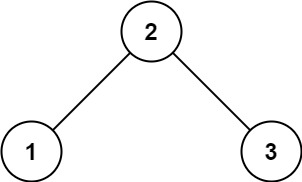| English | 简体中文 |

# 98. Validate Binary Search Tree

## Description

Given the root of a binary tree, determine if it is a valid binary search tree (BST).

A valid BST is defined as follows:

• The left subtree of a node contains only nodes with keys less than the node's key.
• The right subtree of a node contains only nodes with keys greater than the node's key.
• Both the left and right subtrees must also be binary search trees.

Example 1:Input: root = [2,1,3]
Output: true


Example 2:Input: root = [5,1,4,null,null,3,6]
Output: false
Explanation: The root node's value is 5 but its right child's value is 4.


Constraints:

• The number of nodes in the tree is in the range [1, 104].
• -231 <= Node.val <= 231 - 1